# CD Verify Model Type VI

LabVIEW 2018 Control Design and Simulation Module Help

Edition Date: March 2018

Part Number: 371894J-01

»View Product InfoDownload Help (Windows Only)

Owning Palette: Model Information VIs

Requires: Control Design and Simulation Module

Determines the type of system models based on the number of inputs or outputs. A system model can be single-input single-output (SISO), multiple-input multiple-output (MIMO), single-input multiple-output (SIMO), or multiple-input single-output (MISO). Wire data to the State-Space Model input to determine the polymorphic instance to use or manually select the instance.

Use the pull-down menu to select an instance of this VI.

 Select an instance CD Verify Model Type (State-Space)CD Verify Model Type (Transfer Function)CD Verify Model Type (Zero-Pole-Gain)

## CD Verify Model Type (State-Space)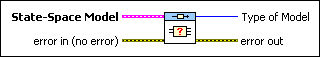State-Space Model contains a mathematical representation of and information about the system for which this VI verifies the model type.error in describes error conditions that occur before this node runs. This input provides standard error in functionality.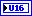Type of Model uses the number of inputs and outputs of the system model to determine if the model is SISO, MIMO, SIMO, or MISO.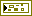error out contains error information. This output provides standard error out functionality.

## CD Verify Model Type (Transfer Function)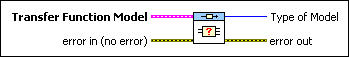Transfer Function Model contains a mathematical representation of and information about the system for which this VI verifies the model type.error in describes error conditions that occur before this node runs. This input provides standard error in functionality.Type of Model uses the number of inputs and outputs of the system model to determine if the model is SISO, MIMO, SIMO, or MISO.error out contains error information. This output provides standard error out functionality.

## CD Verify Model Type (Zero-Pole-Gain)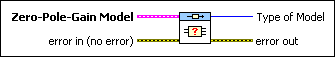Zero-Pole-Gain Model contains a mathematical representation of and information about the system for which this VI verifies the model type.error in describes error conditions that occur before this node runs. This input provides standard error in functionality.Type of Model uses the number of inputs and outputs of the system model to determine if the model is SISO, MIMO, SIMO, or MISO.error out contains error information. This output provides standard error out functionality.